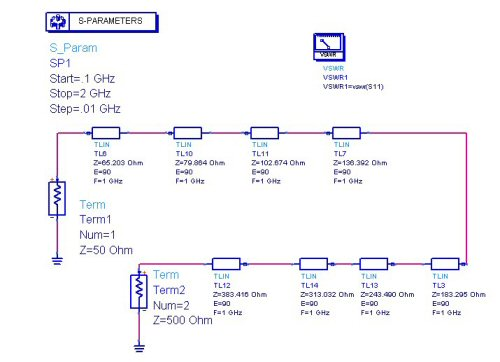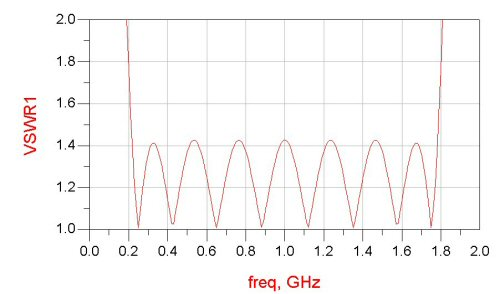# Quarter-wave Transformers

Click here to learn how to calculate Z0 from measured data using transformer theory

Check out our new page on Peter Bramham's twelfth-wave transformer!

New for September 2021: here's an example of an equal-ripple multi-section transformer using a spreadsheet you can download from our site.Transformer Optimus Prime approves this message...

Suppose you want to match a device of a different impedance than your system impedance. A simple quarter-wave transformer can do this for you, with bandwidth somewhat inversely proportional to the relative mismatch you are trying to overcome. For a single-stage quarter-wave transformer, the correct transformer impedance is the geometric mean between the impedances of the load and the source:

ZT=(ZL*ZS)^0.5

We will deal only with purely real impedances here, but transformers can be used successfully to impedance match loads with reactive components as well. The problem is that the math gets ugly quickly. It is doable inside an Excel spreadsheet using complex math. We promise we'll get back to this and post the equations soon (feel free to remind us!)

### Multi-section transformers

There is a natural bandwidth limitation of quarter-wave transformers, because you only get exactly a quarter-wave at one frequency; lower frequencies "see" less than a quarter-wave, higher frequencies see more. However, a second trick is to keep piling quarter-wave transformers in a series, so that the impedance mismatch that each transformer is "correcting" becomes less and less. How do you arrive at the intermediate impedances of the multistage transformer when there are infinite solutions to the problem?

Responses such as Chebyshev (equi-ripple) and maximally flat are possible for multi-section transformers; luckily we have already done the math for you!

### Exponential transformer

First, consider that each each transformer brings you to an intermediate impedance. We can select the intermediate impedances, then solve for the transformer impedances. Below we have transformed 25 ohms to 50 ohms in N=1, N=2, N=3 and N=4 transformers. In each case we have used intermediate impedances in an arithmetic series. For example, for N=4 there are three intermediate impedances. An arithmetic series the steps are equal, so the impedances are 31.25 ohms, 37.5 ohms, 43.75 ohms. Solving for the transformers yields Z1=27.951, Z2=34.233, Z3=40.505 and Z4=46.771 ohms. Return loss of N=1, N=2, N=3 and N=4 transformers matching 50 ohms to 25 ohms are shown below (S11 is for N=1, S22 is for N=2, etc.), in a plot generated using Agilent's Advanced Design System EDA software. The graph illustrates well that bandwidth gets better the more sections we add. However, it is clearly not the best solution to the problem,we would like to see more bandwidth with a -20 dB match, at the expense of the bandwidth that falls below -30 dB.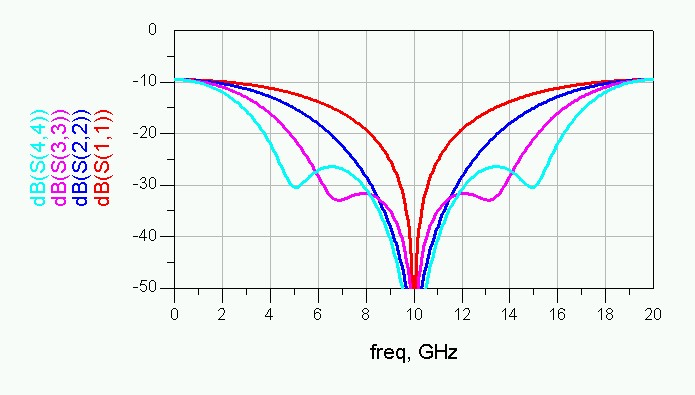Our second "simple solution" to multi-section transformers involves a geometric series from impedance ZL to impedance ZS. Here the impedance from one section to the next adjacent section is always a constant ratio. Plotted below for the same parameters, we like it better than the arithmetic series. Instead of presenting the math here, we offer an Excel file that will calculate up to ten-section transformers for you, it's in the Microwaves101 download area. We didn't even bother to lock the formulas!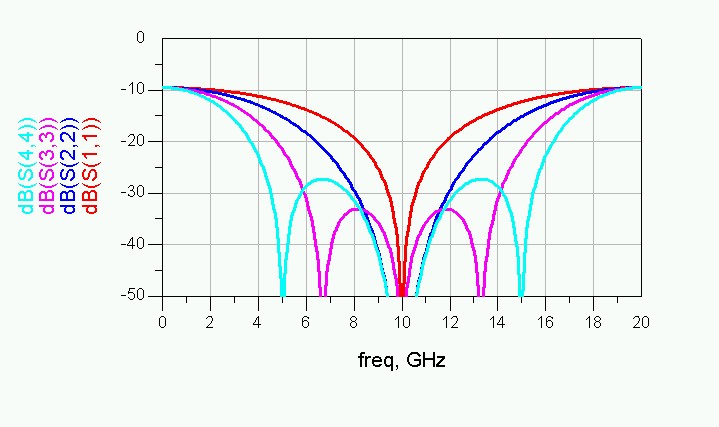So what does a ten-section transformer buy you? Below we have plotted the response of a geometric transformer, 10 sections, matching from 5 to 50 ohms. Here it is apparent that we have failed to provide the familiar Chebyshev equal-ripple response, but our transformer ain't half-bad. What did you want for free?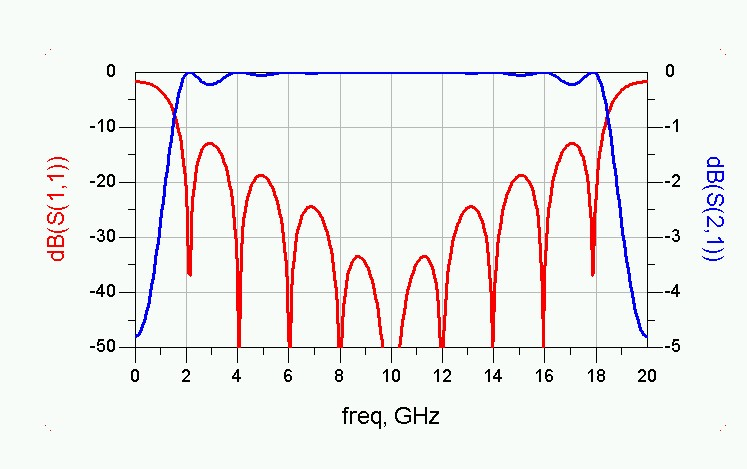### Maximally flat transformers

This topic now has its own page.

### Chebychev (equalt-ripple) transformers

The Chebychev transformer produces equal ripple in passband of your choosing. The maximally-flat and exponential transformers don't allow frequency band as an independent variable. Below is a simple example of a seven-section transformer, but there is a much better example on this page.